Classification Quiz II, Non Verbal Reasoning

# Classification Quiz II, Non Verbal Reasoning - SSC CGL

Test Description

## 20 Questions MCQ Test Non Verbal Reasoning - Classification Quiz II, Non Verbal Reasoning

Classification Quiz II, Non Verbal Reasoning for SSC CGL 2023 is part of Non Verbal Reasoning preparation. The Classification Quiz II, Non Verbal Reasoning questions and answers have been prepared according to the SSC CGL exam syllabus.The Classification Quiz II, Non Verbal Reasoning MCQs are made for SSC CGL 2023 Exam. Find important definitions, questions, notes, meanings, examples, exercises, MCQs and online tests for Classification Quiz II, Non Verbal Reasoning below.
Solutions of Classification Quiz II, Non Verbal Reasoning questions in English are available as part of our Non Verbal Reasoning for SSC CGL & Classification Quiz II, Non Verbal Reasoning solutions in Hindi for Non Verbal Reasoning course. Download more important topics, notes, lectures and mock test series for SSC CGL Exam by signing up for free. Attempt Classification Quiz II, Non Verbal Reasoning | 20 questions in 40 minutes | Mock test for SSC CGL preparation | Free important questions MCQ to study Non Verbal Reasoning for SSC CGL Exam | Download free PDF with solutions
 1 Crore+ students have signed up on EduRev. Have you?
Classification Quiz II, Non Verbal Reasoning - Question 1

### Directions to Solve In each problem, out of the five figures marked (1), (2), (3), (4) and (5), four are similar in a certain manner. However, one figure is not like the other four. Choose the figure which is different from the rest. Question - Choose the figure which is different from the rest.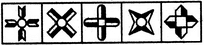(1)     (2)     (3)     (4)     (5)

Detailed Solution for Classification Quiz II, Non Verbal Reasoning - Question 1
All other figures have at least one line of symmetry.
Classification Quiz II, Non Verbal Reasoning - Question 2

### Directions to Solve In each problem, out of the five figures marked (1), (2), (3), (4) and (5), four are similar in a certain manner. However, one figure is not like the other four. Choose the figure which is different from the rest. Question - Choose the figure which is different from the rest.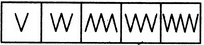(1)     (2)     (3)     (4)     (5)

Detailed Solution for Classification Quiz II, Non Verbal Reasoning - Question 2
Fig. (3) is formed by a combination of A-shaped elements while all other figures are formed by a combination of V-shaped elements.
Classification Quiz II, Non Verbal Reasoning - Question 3

### Directions to Solve In each problem, out of the five figures marked (1), (2), (3), (4) and (5), four are similar in a certain manner. However, one figure is not like the other four. Choose the figure which is different from the rest. Question - Choose the figure which is different from the rest.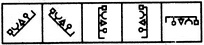(1)     (2)     (3)     (4)     (5)

Detailed Solution for Classification Quiz II, Non Verbal Reasoning - Question 3
All other figures can be rotated into each other.
Classification Quiz II, Non Verbal Reasoning - Question 4

In each problem, out of the five figures marked (1), (2), (3), (4) and (5), four are similar in a certain manner. However, one figure is not like the other four. Choose the figure which is different from the rest.

Question -

Choose the figure which is different from the rest.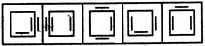(1)     (2)     (3)     (4)     (5)
Detailed Solution for Classification Quiz II, Non Verbal Reasoning - Question 4
In all other figures, the square has two line segments inside and one line segment outside.
Classification Quiz II, Non Verbal Reasoning - Question 5

In each problem, out of the five figures marked (1), (2), (3), (4) and (5), four are similar in a certain manner. However, one figure is not like the other four. Choose the figure which is different from the rest.

Question -

Choose the figure which is different from the rest.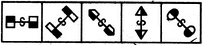(1)     (2)     (3)     (4)     (5)
Detailed Solution for Classification Quiz II, Non Verbal Reasoning - Question 5
Only in fig. (3), the two shaded portions lies on the same side of the main line.
Classification Quiz II, Non Verbal Reasoning - Question 6

In each problem, out of the five figures marked (1), (2), (3), (4) and (5), four are similar in a certain manner. However, one figure is not like the other four. Choose the figure which is different from the rest.

Question -

Choose the figure which is different from the rest.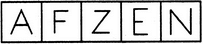(1)     (2)     (3)     (4)     (5)
Detailed Solution for Classification Quiz II, Non Verbal Reasoning - Question 6
Each one of the figures, except fig. (4), is formed by three line segments.
Classification Quiz II, Non Verbal Reasoning - Question 7

In each problem, out of the five figures marked (1), (2), (3), (4) and (5), four are similar in a certain manner. However, one figure is not like the other four. Choose the figure which is different from the rest.

Question -

Choose the figure which is different from the rest.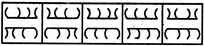(1)     (2)     (3)     (4)     (5)
Detailed Solution for Classification Quiz II, Non Verbal Reasoning - Question 7
In each one of the other four figures, four arcs are curved towards the left and four other acres are curved towards the right.
Classification Quiz II, Non Verbal Reasoning - Question 8

In each problem, out of the five figures marked (1), (2), (3), (4) and (5), four are similar in a certain manner. However, one figure is not like the other four. Choose the figure which is different from the rest.

Question -

Choose the figure which is different from the rest.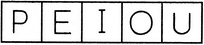(1)     (2)     (3)     (4)     (5)
Detailed Solution for Classification Quiz II, Non Verbal Reasoning - Question 8
All other are vowels.
Classification Quiz II, Non Verbal Reasoning - Question 9

In each problem, out of the five figures marked (1), (2), (3), (4) and (5), four are similar in a certain manner. However, one figure is not like the other four. Choose the figure which is different from the rest.

Question -

Choose the figure which is different from the rest.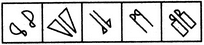(1)     (2)     (3)     (4)     (5)
Detailed Solution for Classification Quiz II, Non Verbal Reasoning - Question 9
All other figures contain a geometrical figure along with its mirror image.
Classification Quiz II, Non Verbal Reasoning - Question 10

In each problem, out of the five figures marked (1), (2), (3), (4) and (5), four are similar in a certain manner. However, one figure is not like the other four. Choose the figure which is different from the rest.

Question -

Choose the figure which is different from the rest.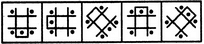(1)     (2)     (3)     (4)     (5)
Detailed Solution for Classification Quiz II, Non Verbal Reasoning - Question 10
All other figures can be rotated into each other.
Classification Quiz II, Non Verbal Reasoning - Question 11

In each problem, out of the five figures marked (1), (2), (3), (4) and (5), four are similar in a certain manner. However, one figure is not like the other four. Choose the figure which is different from the rest.

Question -

Choose the figure which is different from the rest.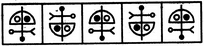(1)     (2)     (3)     (4)     (5)
Detailed Solution for Classification Quiz II, Non Verbal Reasoning - Question 11
All other figures can be rotated into each other.
Classification Quiz II, Non Verbal Reasoning - Question 12

In each problem, out of the five figures marked (1), (2), (3), (4) and (5), four are similar in a certain manner. However, one figure is not like the other four. Choose the figure which is different from the rest.

Question -

Choose the figure which is different from the rest.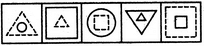(1)     (2)     (3)     (4)     (5)
Detailed Solution for Classification Quiz II, Non Verbal Reasoning - Question 12
In each one of the other figures, one of the two elements is made of dotted lines.
Classification Quiz II, Non Verbal Reasoning - Question 13

In each problem, out of the five figures marked (1), (2), (3), (4) and (5), four are similar in a certain manner. However, one figure is not like the other four. Choose the figure which is different from the rest.

Question -

Choose the figure which is different from the rest.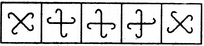(1)     (2)     (3)     (4)     (5)
Detailed Solution for Classification Quiz II, Non Verbal Reasoning - Question 13
All other figures can be rotated into each other.
Classification Quiz II, Non Verbal Reasoning - Question 14

In each problem, out of the five figures marked (1), (2), (3), (4) and (5), four are similar in a certain manner. However, one figure is not like the other four. Choose the figure which is different from the rest.

Question -

Choose the figure which is different from the rest.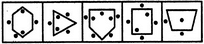(1)     (2)     (3)     (4)     (5)
Detailed Solution for Classification Quiz II, Non Verbal Reasoning - Question 14
In all other figures, the number of dots outside the main figure is one more than the number of dots inside the main figure.
Classification Quiz II, Non Verbal Reasoning - Question 15

In each problem, out of the five figures marked (1), (2), (3), (4) and (5), four are similar in a certain manner. However, one figure is not like the other four. Choose the figure which is different from the rest.

Question -

Choose the figure which is different from the rest.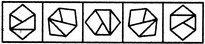(1)     (2)     (3)     (4)     (5)
Detailed Solution for Classification Quiz II, Non Verbal Reasoning - Question 15
All other figures can be rotated into each other.
Classification Quiz II, Non Verbal Reasoning - Question 16

In each problem, out of the five figures marked (1), (2), (3), (4) and (5), four are similar in a certain manner. However, one figure is not like the other four. Choose the figure which is different from the rest.

Question -

Choose the figure which is different from the rest.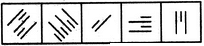(1)     (2)     (3)     (4)     (5)
Detailed Solution for Classification Quiz II, Non Verbal Reasoning - Question 16
In all other figures, all the line segments are drawn perpendicular to one base only.
Classification Quiz II, Non Verbal Reasoning - Question 17

In each problem, out of the five figures marked (1), (2), (3), (4) and (5), four are similar in a certain manner. However, one figure is not like the other four. Choose the figure which is different from the rest.

Question -

Choose the figure which is different from the rest.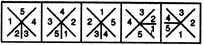(1)     (2)     (3)     (4)     (5)
Detailed Solution for Classification Quiz II, Non Verbal Reasoning - Question 17
Only in fig. (2), while moving in an ACW direction, the numbers do not form a sequence.
Classification Quiz II, Non Verbal Reasoning - Question 18

In each problem, out of the five figures marked (1), (2), (3), (4) and (5), four are similar in a certain manner. However, one figure is not like the other four. Choose the figure which is different from the rest.

Question -

Choose the figure which is different from the rest.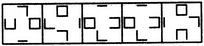(1)     (2)     (3)     (4)     (5)
Detailed Solution for Classification Quiz II, Non Verbal Reasoning - Question 18
Each one of the figures except fig. (2), contains - one complete square, one cup-shaped element having three sides, one 'U-shaped element having two sides and one straight line.
Classification Quiz II, Non Verbal Reasoning - Question 19

In each problem, out of the five figures marked (1), (2), (3), (4) and (5), four are similar in a certain manner. However, one figure is not like the other four. Choose the figure which is different from the rest.

Question -

Choose the figure which is different from the rest.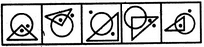(1)     (2)     (3)     (4)     (5)
Detailed Solution for Classification Quiz II, Non Verbal Reasoning - Question 19
In all other figures, one of the dots lies outside the triangle as well as the circle.
Classification Quiz II, Non Verbal Reasoning - Question 20

In each problem, out of the five figures marked (1), (2), (3), (4) and (5), four are similar in a certain manner. However, one figure is not like the other four. Choose the figure which is different from the rest.

Question -

Choose the figure which is different from the rest.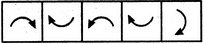(1)     (2)     (3)     (4)     (5)
Detailed Solution for Classification Quiz II, Non Verbal Reasoning - Question 20
In fig. (3), the arrow indicates an ACW motion while in each of the other figures, the arrow indicates a CW motion.

## Non Verbal Reasoning

40 tests
Information about Classification Quiz II, Non Verbal Reasoning Page
In this test you can find the Exam questions for Classification Quiz II, Non Verbal Reasoning solved & explained in the simplest way possible. Besides giving Questions and answers for Classification Quiz II, Non Verbal Reasoning, EduRev gives you an ample number of Online tests for practice

40 tests